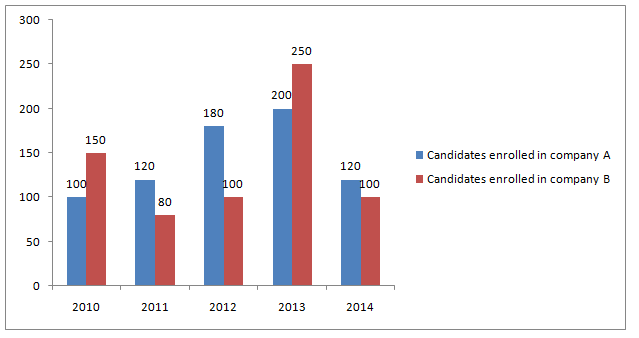# RRB PO Prelims Quantitative Aptitude Questions 2021 (Day-07)

Dear Aspirants, Our IBPS Guide team is providing new series of Quantitative Aptitude Questions for RRB PO Prelims 2021 so the aspirants can practice it on a daily basis. These questions are framed by our skilled experts after understanding your needs thoroughly. Aspirants can practice these new series questions daily to familiarize with the exact exam pattern and make your preparation effective.

Start Quiz

Wrong number series

Directions (01-05): Find out the wrong number in the following number series.

1) 13, 26, 78, 312, 1560, 9380

A.13

B.26

C.312

D.9380

E.1560

2) 8, 19, 35, 56, 82, 111

A.111

B.56

C.19

D.82

E.35

3) 67, 132, 183, 222, 251, 275

A.251

B.222

C.275

D.183

E.132

4) 19, 21, 33, 73, 188, 473

A.21

B.188

C.33

D.473

E.73

5) 13, 23, 41, 65, 101, 143

A.65

B.101

C.23

D.143

E.41

Bar graph

Directions (06-10): Study the following information carefully and answer the questions given below.

The bar graph shows the total number of candidates enrolled in different years from 2010 to 2014 in two companies A and B.6) If in the year 2015, there is 20% increase in the total number of candidates enrolled in 2014 in both the companies, then find the total number of candidates enrolled in 2015?

A.232

B.240

C.264

D.280

E.None of these

7) The number of candidates enrolled in company A in 2010 is what percentage of the number of candidates enrolled in company B in 2013?

A.20%

B.40%

C.25%

D.30%

E.None of these

8) The total number of candidates enrolled in company A in 2012 and 2013 together is what percentage more than the number of candidates enrolled in company B in 2010 and 2011 together?

A.50.12%

B.65.21%

C.78.12%

D.42.18%

E.54.81%

9) What is the ratio of the total number of candidates in both companies in 2010 to the total number of candidates in 2013 of both companies?

A.2: 3

B.3: 2

C.9: 5

D.5: 9

E.None of these

10) The number of candidates enrolled in company A from 2012 to 2014 together is what percentage more than the number of candidates enrolled in company B in 2010 and 2011 together?(approx..)

A.190%

B.98%

C.140%

D.130%

E.117%

13 * 2 = 26

26 * 3 = 78

78 * 4 = 312

312 * 5 = 1560

1560 * 6 = 9360

8 + (11 + 0) = 19

19 + (11 + 5) = 35

35 + (11 + 10) = 56

56 + (11 + 15) = 82

82 + (11 + 20) = 113

67 + 82 + 1 = 132

132 + 72 + 2 = 183

183 + 62 + 3 = 222

222 + 52 + 4 = 251

251 + 42 + 5 = 272

19 + (1 * 2) = 21

21 + (3 * 4) = 33

33 + (5 * 8) = 73

73 + (7 * 16) = 185

185 + (9 * 32) = 473

13 + 10 = 23

23 + 18 = 41

41 + 26 = 67

67 + 34 = 101

101 + 42 = 143

Total number of candidates enrolled in 2015 = 120/100 * (120 + 100)

= 264

Required % = (number of candidates enrolled in A in 2010/number of candidates enrolled in B in 2013) * 100

= 100/250 * 100 = 40%

Total number of candidates enrolled in company A in 2012 and 2013 = 180 + 200= 380

Total number of candidates enrolled in company B in 2010 and 2011 = 150 + 80= 230

Required % = (380 – 230)/230 * 100 = 65.21%

Total number of candidates enrolled in 2010 = 100 + 150 = 250

Total number of candidates enrolled in 2013 = 200 + 250 = 450

Required ratio = 250: 450 = 5: 9

Number of candidates enrolled in company A from 2012 to 2014 = 180 + 200 + 120 = 500

Number of candidates enrolled in company B in 2010 and 2011 = 150 + 80 = 230

Required % = (500 – 230)/230 * 100

= 117.39% ≈ 117%

1 1 vote
Rating